# SAT Math Multiple Choice Question 989: Answer and Explanation

### Test Information

Question: 989

9.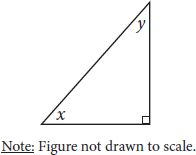In the right triangle above, cos x = 0.8. What is the value of cos y?

• A.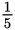• B.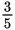• C.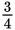• D.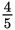Explanation:

B

Difficulty: Easy

Category: Additional Topics in Math / Trigonometry

Strategic Advice: Whenever trig values are given as decimal numbers, convert them to fractions so you can use the ratios given by SOH CAH TOA.

Getting to the Answer: The decimal 0.8 equals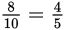, which should make you think of a 3:4:5 triangle. Indeed, that's exactly what this triangle is. Draw a quick sketch and use SOH CAH TOA to place the side lengths in the correct places, ensuring that cos x =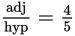. That is, label the side adjacent to angle x as 4 and the hypotenuse 5. The remaining side must have length 3.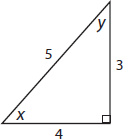Again by SOH CAH TOA (and looking at angle y this time), cos y =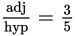, choice (B).